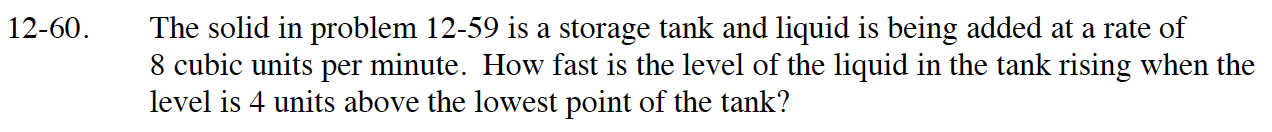### Home > CALC > Chapter Ch12 > Lesson 12.1.5 > Problem12-60

12-60.Graph the functions.
The lowest point is at y = 4.
The height is y. The radius is x.
The volume will be πx2dy.

Express the main function in terms of x, which is the radius.

$V=\int_4^{h+4}\pi\Big(\frac{y^2}{16}-1\Big)^{4/3}dy$

$\frac{dV}{dt}=\frac{d}{dt}\int_4^{h+4}\pi\Big(\frac{y^2}{16}-1\Big)^{4/3}dy$

$8=\pi\Big(\frac{(h+4)^2}{16}-1\Big)^{4/3}\cdot\frac{dh}{dt}$

Let h = 4 and solve for dh/dt.